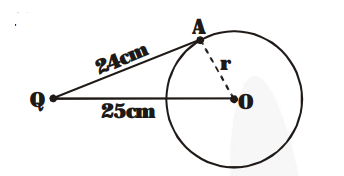# From a point Q,

Question:

From a point Q, the length of the tangent to a circle is 24 cm and the distance of Q from the centre is 25 cm. The radius of the circle is -

(A) 7 cm

(B) 12 cm

(C) 15 cm

(D) 24.5 cm

Solution:

From figure,$r^{2}=(25)^{2}-(24)^{2}$

$=625-576$

$=49$

$\Rightarrow \mathrm{r}=7 \mathrm{~cm}$

Hence, the correct option is (A)